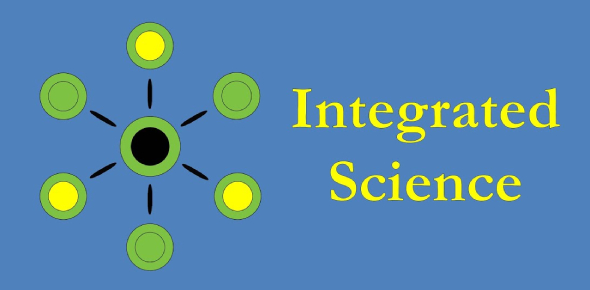# Practice Integrated Science Quiz

14 Questions | Total Attempts: 4425Settings.

• 1.
About how many atomic numbers are there?
• A.

100

• B.

1000

• C.

10000

• 2.
About how many compounds are there?
• A.

• B.

• C.

Far greater than 10,000

• 3.
Which of the following are organic compounds?
• A.

NaCl

• B.

C6H12O6

• C.

C2H4

• D.

H2O

• 4.
Minerals are produced...
• A.

In the Stomach

• B.

In the ocean

• C.

None of the above

• 5.
A proton ....
• A.

Has a 2+ charge

• B.

Is outside of the nucleus

• C.

Has a negative charge

• D.

None of the above

• 6.
All atoms of the same elements have the same:
• A.

Number of protons

• B.

Number of molecules

• C.

Atomic weight

• D.

Number of electrons

• 7.
A hypothesis is less supported than a theory.
• A.

True

• B.

False

• 8.
A scientific theory is always absolute.
• A.

True

• B.

False

• 9.
What are the 6 most commonly used elements in chemistry?
• A.

C

• B.

Br

• C.

H

• D.

S

• E.

P

• F.

N

• G.

Zr

• H.

O

• 10.
Ions are...
• A.

Long distances

• B.

Charged Particles

• C.

Orbitals

• 11.
The polarity of water can cause hydrogen molecules to bond
• A.

True

• B.

False

• 12.
An atom becomes an ion when it
• A.

Loses it's nucleus

• B.

Gains or loses electrons

• C.

Bonds to an isotope

• 13.
The compound CO2 represents
• A.

Two atoms of carbon and two atoms of oxygen

• B.

One molecule of carbon and two molecules of oxygen

• C.

One atom of carbon and two atoms of oxygen

• 14.
Protons move at a very fast pace/speed around the outside of the nucleus.
• A.

True

• B.

False

Related TopicsBack to top